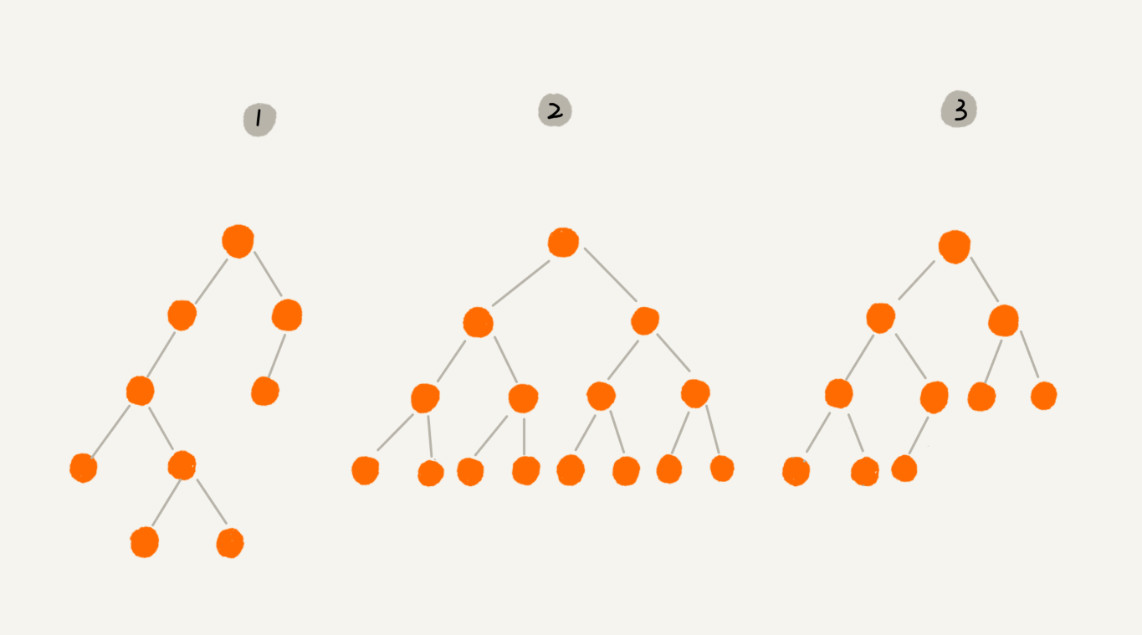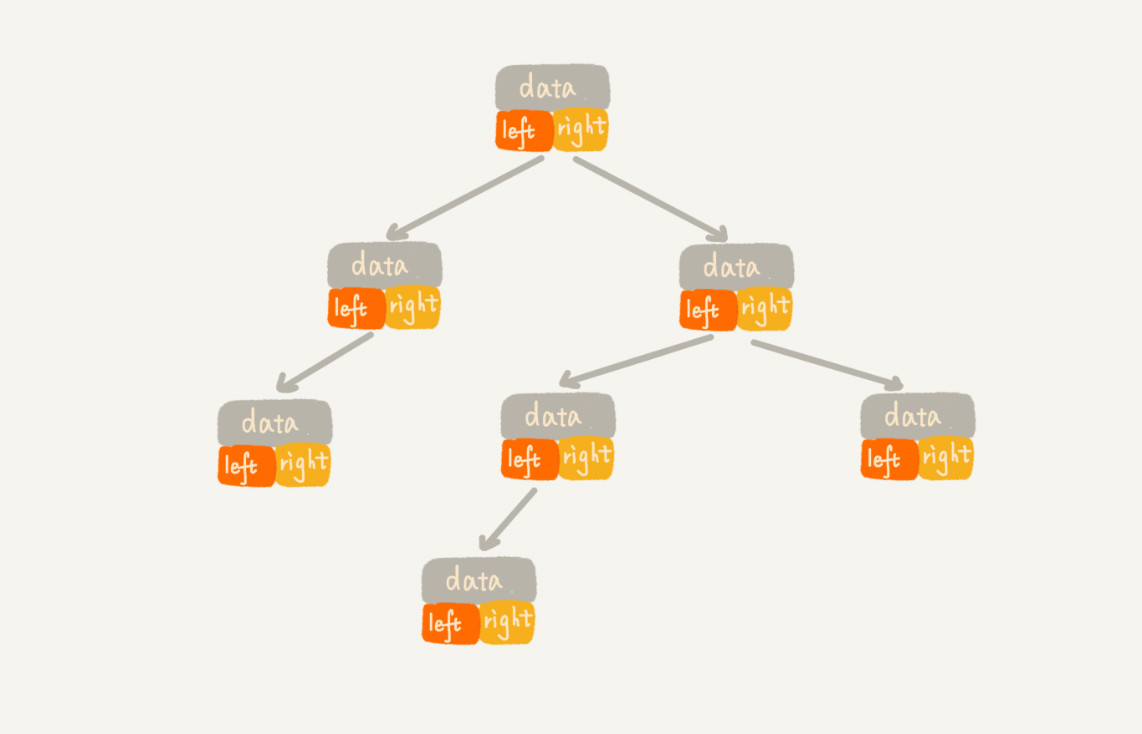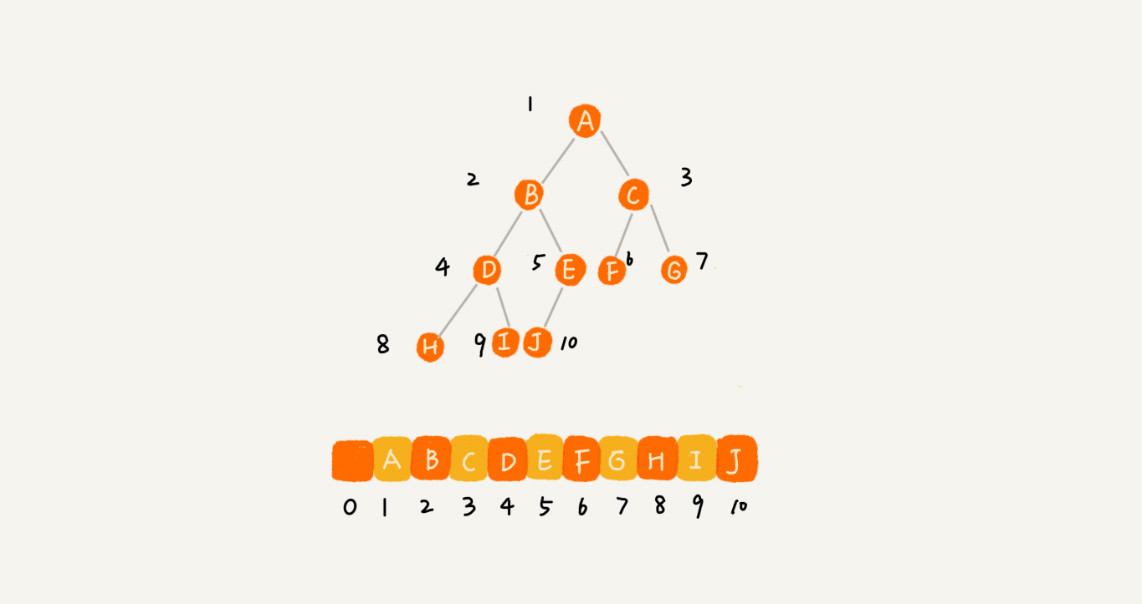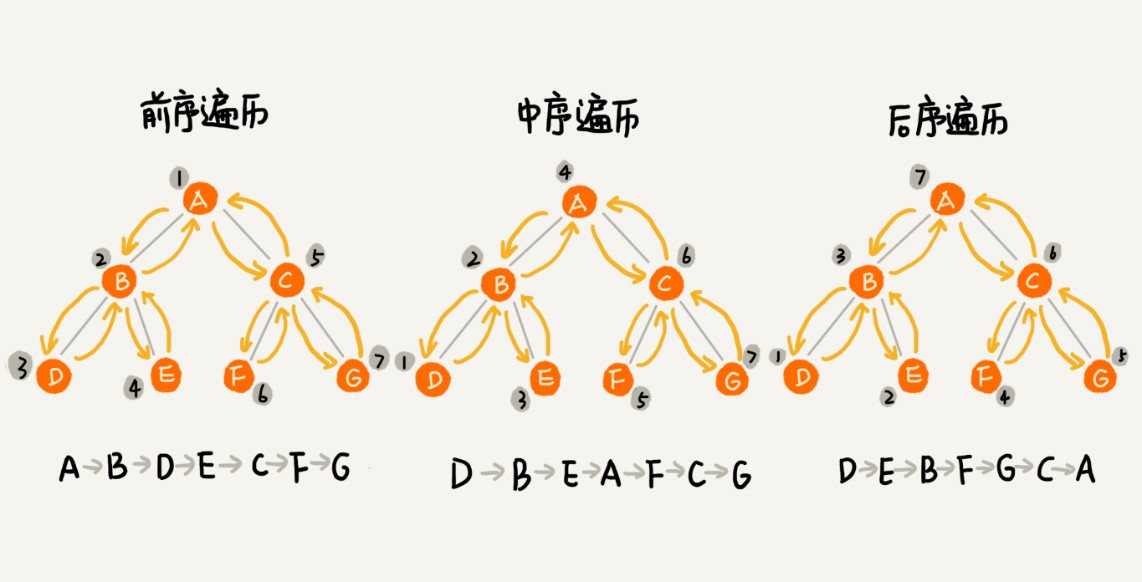# 1. 树

• 父节点
• 上层的节点
• 兄弟节点
• 父节点是同一个节点
• 叶节点
• 没有子节点的节点
• 高度
• 节点到叶子节点的最长路径
• 深度
• 根节点到这个节点所经历的边的个数
• 节点的深度 + 1

# 2. 二叉树2 显示的是满二叉树，特点是叶子节点都在最底层，出了叶子节点之外，每个节点都有左右两个子节点

3 叶子节点都在最底下两层，并且出了最后一层，其他的层的节点数量都要达到最大，并且最后一层的节点都是靠左排列的，这种二叉树叫做完全二叉树。

## 2.1 如何表示/ 存储一棵二叉树？

### 2.1.1 基于指针或者引用的二叉链式存储法### 2.1.2 基于数组的顺序存储法## 2.2 二叉树的遍历

### 2.2.0 递归公式``````前序遍历的递推公式：
preOrder(r) = print r->preOrder(r->left)->preOrder(r->right)

inOrder(r) = inOrder(r->left)->print r->inOrder(r->right)

postOrder(r) = postOrder(r->left)->postOrder(r->right)->print r

// 前序遍历
void preOrder(Node* root) {
if (root == null) return;
print root // 此处为伪代码，表示打印root节点
preOrder(root->left);
preOrder(root->right);
}

// 中序遍历
void inOrder(Node* root) {
if (root == null) return;
inOrder(root->left);
print root // 此处为伪代码，表示打印root节点
inOrder(root->right);
}

// 后序遍历
void postOrder(Node* root) {
if (root == null) return;
postOrder(root->left);
postOrder(root->right);
print root // 此处为伪代码，表示打印root节点
}``````

# 3. 二叉查找树

×